• ## matlab图像处理常用函数大全

万次阅读 多人点赞 2018-07-16 16:03:34
显示索引图像和灰度图像

>> gmap=rgb2gray(map);

>> figure,imshow(X,map);

>> figure,imshow(X,gmap);

利用膨胀函数平移图像

se = translate(strel(1), [30 30]);%将一个平面结构化元素分别向下和向右移动30个位置

J = imdilate(I,se);%利用膨胀函数平移图像

subplot(121);imshow(I), title('原图')

subplot(122), imshow(J), title('移动后的图像');

水平翻转和上下翻转

Flip1=fliplr(I);               %  对矩阵I左右反转

subplot(131);imshow(I);title('原图');

subplot(132);imshow(Flip1);title('水平镜像');

Flip2=flipud(I);               %  对矩阵I垂直反转

subplot(133);imshow(Flip2);title('竖直镜像');

图像旋转

B=imrotate(I,60,'bilinear','crop');

%双线性插值法旋转图像，并裁剪图像，使其和原图像大小一致

subplot(121),imshow(I),title('原图');

subplot(122),imshow(B),title('旋转图像60^{o}，并剪切图像');

截取图像

I2 = imcrop(I,[75 68 130 112]);

imshow(I), figure, imshow(I2)

画轮廓

>> imshow(I)

>> figure

>> imcontour(I,3)

噪声和滤波

J=imnoise(I,'salt & pepper',0.02);%添加椒盐噪声

subplot(121),imshow(J);title('噪声图像')

K=medfilt2(J);%使用3*3的邻域窗的中值滤波

subplot(122),imshow(K);title('中值滤波后图像')

Matlab数字数字图像处理函数汇总：

1、数字数字图像的变换

j=fft2(i);

②ifft2:：ifft2函数用于数字数字图像的二维傅立叶反变换，如：

j=fft2(i);

k=ifft2(j);

2、模拟噪声生成函数和预定义滤波器

① imnoise：用于对数字数字图像生成模拟噪声，如：

j=imnoise(i,'gaussian',0,0.02);%模拟高斯噪声

② fspecial：用于产生预定义滤波器，如：

h=fspecial('sobel');%sobel水平边缘增强滤波器

h=fspecial('gaussian');%高斯低通滤波器

h=fspecial('laplacian');%拉普拉斯滤波器

h=fspecial('log');%高斯拉普拉斯（LoG）滤波器

h=fspecial('average');%均值滤波器

2、数字数字图像的增强

①直方图：imhist函数用于数字数字图像的直方图显示，如：

imhist(i);

②直方图均化：histeq函数用于数字数字图像的直方图均化，如：

j=histeq(i);

imshow(J)

④对数变换：log函数用于数字数字图像的对数变换，如：

j=double(i);

k=log(j);

h=[1,2,1;0,0,0;-1,-2,-1];

j=filter2(h,i);

⑥线性滤波：利用二维卷积conv2滤波, 如:

h=[1,1,1;1,1,1;1,1,1];

h=h/9;

j=conv2(i,h);

⑦中值滤波：medfilt2函数用于数字数字图像的中值滤波，如：

j=medfilt2(i);

⑧锐化

（1）利用Sobel算子锐化数字数字图像, 如:

h=[1,2,1;0,0,0;-1,-2,-1];%Sobel算子

j=filter2(h,i);

（2）利用拉氏算子锐化数字数字图像, 如:

j=double(i);

h=[0,1,0;1,-4,0;0,1,0];%拉氏算子

k=conv2(j,h,'same');

m=j-k;

3、数字数字图像边缘检测

①sobel算子 如：

j =edge(i,'sobel',thresh)

②prewitt算子 如：

j =edge(i,'prewitt',thresh)

③roberts算子  如：

j =edge(i,'roberts',thresh)

④log算子  如：

j =edge(i,'log',thresh)

⑤canny算子 如：

j =edge(i,'canny',thresh)

⑥Zero-Cross算子 如：

j =edge(i,'zerocross',thresh)

4、形态学数字数字图像处理

①膨胀：是在二值化数字数字图像中“加长”或“变粗”的操作，函数imdilate执行膨胀运算，如：

b=[0 1 0;1 1 1;01 0];

c=imdilate(a,b);

②腐蚀：函数imerode执行腐蚀，如：

b=strel('disk',1);

c=imerode(a,b);

③开运算：先腐蚀后膨胀称为开运算，用imopen来实现，如：

b=strel('square',2);

c=imopen(a,b);

④闭运算：先膨胀后腐蚀称为闭运算，用imclose来实现，如：

b=strel('square',2);

c=imclose(a,b);

数字数字图像增强

1. 直方图均衡化的 Matlab 实现

1.1 imhist 函数

功能：计算和显示数字数字图像的色彩直方图

格式：imhist(I,n)

imhist(X,map)

说明：imhist(I,n) 其中，n 为指定的灰度级数目，缺省值为256；imhist(X,map) 就算和显示索引色数字数字图像 X 的直方图，map为调色板。用stem(x,counts) 同样可以显示直方图。

1.2 imcontour 函数

功能：显示数字数字图像的等灰度值图

格式：imcontour(I,n),imcontour(I,v)

说明：n 为灰度级的个数，v 是有用户指定所选的等灰度级向量。

功能：通过直方图变换调整对比度

说明：J=imadjust(I,[low high],[bottomtop],gamma) 其中，gamma 为校正量r，[lowhigh] 为原数字数字图像中要变换的灰度范围，[bottom top]

指定了变换后的灰度范围；newmap=imadjust(map,[lowhigh],[bottom top],gamma) 调整索引色数字数字图像的调色板 map 。此时若 [low high] 和

[bottom top] 都为2×3的矩阵，则分别调整 R、G、B 3个分量。

1.4 histeq 函数

功能：直方图均衡化

格式：J=histeq(I,hgram)

J=histeq(I,n)

[J,T]=histeq(I,...)

newmap=histeq(X,map,hgram)

newmap=histeq(X,map)

[new,T]=histeq(X,...)

说明：J=histeq(I,hgram) 实现了所谓“直方图规定化”，即将原是图象 I 的直方图变换成用户指定的向量 hgram 。hgram 中的每一个元素

都在 [0,1] 中；J=histeq(I,n) 指定均衡化后的灰度级数 n ，缺省值为 64；[J,T]=histeq(I,...)返回从能将数字数字图像 I 的灰度直方图变换成

数字数字图像 J 的直方图的变换 T ；newmap=histeq(X,map) 和 [new,T]=histeq(X,...) 是针对索引色数字数字图像调色板的直方图均衡。

2. 噪声及其噪声的 Matlab 实现

imnoise 函数

格式：J=imnoise(I,type)

J=imnoise(I,type,parameter)

说明：J=imnoise(I,type) 返回对数字数字图像 I 添加典型噪声后的有噪数字数字图像 J ，参数type 和 parameter 用于确定噪声的类型和相应的参数。

3. 数字数字图像滤波的 Matlab 实现

3.1 conv2 函数

功能：计算二维卷积

格式：C=conv2(A,B)

C=conv2(Hcol,Hrow,A)

C=conv2(...,'shape')

说明：对于 C=conv2(A,B) ，conv2 的算矩阵A 和 B 的卷积，若[Ma,Na]＝size(A), [Mb,Nb]=size(B), 则 size(C)=[Ma+Mb-1,Na+Nb-1];

C=conv2(Hcol,Hrow,A) 中，矩阵 A 分别与Hcol 向量在列方向和 Hrow 向量在行方向上进行卷积；C=conv2(...,'shape') 用来指定 conv2

返回二维卷积结果部分，参数 shape 可取值如下：

》full为缺省值，返回二维卷积的全部结果；

》same返回二维卷积结果中与 A 大小相同的中间部分；

valid 返回在卷积过程中，未使用边缘补 0 部分进行计算的卷积结果部分，当 size(A)>size(B) 时，size(C)=[Ma-Mb+1,Na-Nb+1]。

3.2 conv 函数

功能：计算多维卷积

格式：与 conv2 函数相同

3.3 filter2函数

功能：计算二维线型数字滤波，它与函数 fspecial 连用

格式：Y=filter2(B,X)

Y=filter2(B,X,'shape')

说明：对于 Y=filter2(B,X) ，filter2 使用矩阵B 中的二维 FIR 滤波器对数据 X 进行滤波，结果 Y 是通过二维互相关计算出来的，其大

小与 X 一样；对于Y=filter2(B,X,'shape') ，filter2返回的 Y 是通过二维互相关计算出来的，其大小由参数 shape 确定，其取值如下

：

》full返回二维相关的全部结果，size(Y)>size(X)；

》same返回二维互相关结果的中间部分，Y 与X 大小相同；

》valid返回在二维互相关过程中，未使用边缘补 0 部分进行计算的结果部分，有 size(Y)<size(X) 。

3.4 fspecial 函数

功能：产生预定义滤波器

格式：H=fspecial(type)

H=fspecial('gaussian',n,sigma)        高斯低通滤波器

H=fspecial('sobel')                        Sobel 水平边缘增强滤波器

H=fspecial('prewitt')                     Prewitt 水平边缘增强滤波器

H=fspecial('laplacian',alpha)            近似二维拉普拉斯运算滤波器

H=fspecial('log',n,sigma)                高斯拉普拉斯（LoG）运算滤波器

H=fspecial('average',n)                  均值滤波器

H=fspecial('unsharp',alpha)            模糊对比增强滤波器

说明：对于形式 H=fspecial(type) ，fspecial 函数产生一个由 type 指定的二维滤波器 H ，返回的H 常与其它滤波器搭配使用。

4. 彩色增强的 Matlab 实现

4.1 imfilter函数

功能：真彩色增强

格式：B=imfilter(A,h)

说明：将原始数字数字图像 A 按指定的滤波器 h 进行滤波增强处理，增强后的数字数字图像 B 与A 的尺寸和类型相同

数字数字图像的变换

1. 离散傅立叶变换的Matlab 实现

Matlab 函数 fft、fft2 和 fftn 分别可以实现一维、二维和 N 维 DFT 算法；而函数 ifft、ifft2 和 ifftn 则用来计算反 DFT 。

这些函数的调用格式如下：

A＝fft(X,N,DIM)

其中，X 表示输入数字数字图像；N 表示采样间隔点，如果 X 小于该数值，那么 Matlab 将会对 X 进行零填充，否则将进行截取，使之长度为

N ；DIM 表示要进行离散傅立叶变换。

A＝fft2(X,MROWS,NCOLS)

其中，MROWS 和 NCOLS 指定对 X 进行零填充后的 X 大小。

A＝fftn(X,SIZE)

其中，SIZE 是一个向量，它们每一个元素都将指定 X 相应维进行零填充后的长度。

函数 ifft、ifft2 和 ifftn的调用格式于对应的离散傅立叶变换函数一致。

例子：数字数字图像的二维傅立叶频谱

% 读入原始数字数字图像

imshow(I)

% 求离散傅立叶频谱

J=fftshift(fft2(I));

figure;

imshow(log(abs(J)),[8,10])

2. 离散余弦变换的 Matlab实现

2.1. dCT2 函数

功能：二维 DCT 变换

格式：B=dct2(A)

B=dct2(A,m,n)

B=dct2(A,[m,n])

说明：B＝dct2(A) 计算 A 的 DCT 变换 B ，A 与 B 的大小相同；B＝dct2(A,m,n) 和 B=dct2(A,[m,n]) 通过对 A 补 0 或剪裁，使 B 的大小为 m×n。

2.2. dict2 函数

功能：DCT 反变换

格式：B=idct2(A)

B=idct2(A,m,n)

B=idct2(A,[m,n])

说明：B＝idct2(A) 计算 A 的 DCT 反变换 B ，A 与 B 的大小相同；B＝idct2(A,m,n) 和 B=idct2(A,[m,n]) 通过对 A 补 0 或剪裁，使 B的大小为 m×n。

2.3. dctmtx函数

功能：计算 DCT 变换矩阵

格式：D＝dctmtx(n)

说明：D＝dctmtx(n) 返回一个 n×n 的 DCT 变换矩阵，输出矩阵 D 为 double 类型。

3. 数字数字图像小波变换的Matlab 实现

3.1 一维小波变换的 Matlab实现

(1) dwt 函数

功能：一维离散小波变换

格式：[cA,cD]=dwt(X,'wname')

[cA,cD]=dwt(X,Lo_D,Hi_D)

说明：[cA,cD]=dwt(X,'wname')使用指定的小波基函数'wname' 对信号 X 进行分解，cA、cD分别为近似分量和细节分量；[cA,cD]=dwt(X,Lo_D,Hi_D) 使用指定的滤波器组 Lo_D、Hi_D 对信号进行分解。

(2) idwt 函数

功能：一维离散小波反变换

格式：X=idwt(cA,cD,'wname')

X=idwt(cA,cD,Lo_R,Hi_R)

X=idwt(cA,cD,'wname',L)

X=idwt(cA,cD,Lo_R,Hi_R,L)

说明：X=idwt(cA,cD,'wname')由近似分量 cA 和细节分量 cD 经小波反变换重构原始信号 X 。

'wname' 为所选的小波函数

X=idwt(cA,cD,Lo_R,Hi_R) 用指定的重构滤波器 Lo_R 和 Hi_R 经小波反变换重构原始信号 X 。

X=idwt(cA,cD,'wname',L) 和 X=idwt(cA,cD,Lo_R,Hi_R,L) 指定返回信号 X 中心附近的 L 个点。

3.2 二维小波变换的 Matlab实现

二维小波变换的函数

-------------------------------------------------

函数名                函数功能

---------------------------------------------------

dwt2           二维离散小波变换

wavedec2       二维信号的多层小波分解

idwt2          二维离散小波反变换

waverec2        二维信号的多层小波重构

wrcoef2          由多层小波分解重构某一层的分解信号

upcoef2          由多层小波分解重构近似分量或细节分量

detcoef2         提取二维信号小波分解的细节分量

appcoef2        提取二维信号小波分解的近似分量

upwlev2         二维小波分解的单层重构

dwtpet2         二维周期小波变换

idwtper2        二维周期小波反变换

-------------------------------------------------------------

(1) wcodemat 函数

功能：对数据矩阵进行伪彩色编码

格式：Y=wcodemat(X,NB,OPT,ABSOL)

Y=wcodemat(X,NB,OPT)

Y=wcodemat(X,NB)

Y=wcodemat(X)

说明：Y=wcodemat(X,NB,OPT,ABSOL)返回数据矩阵 X 的编码矩阵 Y ；NB 伪编码的最大值，即编码范围为 0～NB，缺省值 NB＝16；

OPT 指定了编码的方式（缺省值为 'mat'），即：

OPT＝'row' ，按行编码

OPT＝'col' ，按列编码

OPT＝'mat' ，按整个矩阵编码

ABSOL 是函数的控制参数（缺省值为 '1'），即：

ABSOL＝0 时，返回编码矩阵

ABSOL＝1 时，返回数据矩阵的绝对值ABS(X)

(2) dwt2 函数

功能：二维离散小波变换

格式：[cA,cH,cV,cD]=dwt2(X,'wname')

[cA,cH,cV,cD]=dwt2(X,Lo_D,Hi_D)

说明：[cA,cH,cV,cD]=dwt2(X,'wname')使用指定的小波基函数 'wname' 对二维信号 X 进行二维离散小波变幻；cA，cH,cV,cD 分别为近似分

量、水平细节分量、垂直细节分量和对角细节分量；[cA,cH,cV,cD]=dwt2(X,Lo_D,Hi_D) 使用指定的分解低通和高通滤波器 Lo_D 和 Hi_D 分

解信号 X 。

(3) wavedec2 函数

功能：二维信号的多层小波分解

格式：[C,S]=wavedec2(X,N,'wname')

[C,S]=wavedec2(X,N,Lo_D,Hi_D)

说明：[C,S]=wavedec2(X,N,'wname')使用小波基函数'wname' 对二维信号 X 进行 N 层分解；[C,S]=wavedec2(X,N,Lo_D,Hi_D) 使用指定

的分解低通和高通滤波器Lo_D 和 Hi_D 分解信号 X 。

(4) idwt2 函数

功能：二维离散小波反变换

格式：X=idwt2(cA,cH,cV,cD,'wname')

X=idwt2(cA,cH,cV,cD,Lo_R,Hi_R)

X=idwt2(cA,cH,cV,cD,'wname',S)

X=idwt2(cA,cH,cV,cD,Lo_R,Hi_R,S)

说明：X=idwt2(cA,cH,cV,cD,'wname')由信号小波分解的近似信号cA 和细节信号 cH、cH、cV、cD 经小波反变换重构原信号 X

；X=idwt2(cA,cH,cV,cD,Lo_R,Hi_R)使用指定的重构低通和高通滤波器 Lo_R 和 Hi_R 重构原信号 X ；X=idwt2(cA,cH,cV,cD,'wname',S)

和X=idwt2(cA,cH,cV,cD,Lo_R,Hi_R,S) 返回中心附近的 S 个数据点。

(5) waverec2 函数

说明：二维信号的多层小波重构

格式：X=waverec2(C,S,'wname')

X=waverec2(C,S,Lo_R,Hi_R)

说明：X=waverec2(C,S,'wname')由多层二维小波分解的结果 C、S 重构原始信号 X ，'wname'为使用的小波基函数；X=waverec2(C,S,Lo_R,Hi_R) 使用重构低通和高通滤波器 Lo_R 和 Hi_R 重构原信号。

数字数字图像处理工具箱

1. 数字数字图像和数字数字图像数据

缺省情况下，MATLAB将数字数字图像中的数据存储为双精度类型(double)，64位浮点

数，所需存储量很大；MATLAB还支持另一种类型无符号整型(uint8)，即数字数字图像矩

阵中每个数据占用1个字节。

在使用MATLAB工具箱时，一定要注意函数所要求的参数类型。另外，uint8

与double两种类型数据的值域不同，编程需注意值域转换。

从uint8到double的转换

---------------------------------------------

数字数字图像类型        MATLAB语句

---------------------------------------------

索引色             B=double(A)+1

索引色或真彩色 B=double(A)/255

二值数字数字图像          B=double(A)

---------------------------------------------

从double到uint8的转换

---------------------------------------------

数字数字图像类型        MATLAB语句

---------------------------------------------

索引色              B=uint8(round(A-1))

索引色或真彩色   B=uint8(round(A*255))

二值数字数字图像           B=logical(uint8(round(A)))

---------------------------------------------

2. 数字数字图像处理工具箱所支持的数字数字图像类型

2.1 真彩色数字数字图像

R、G、B三个分量表示一个像素的颜色。如果要读取数字数字图像中(100,50)处的像素值，

可查看三元数据(100,50,1:3)。

真彩色数字数字图像可用双精度存储，亮度值范围是[0,1]；比较符合习惯的存储方法是用无

符号整型存储，亮度值范围[0,255]

2.2 索引色数字数字图像

包含两个结构，一个是调色板，另一个是数字数字图像数据矩阵。调色板是一个有3列和若干行

的色彩映象矩阵，矩阵每行代表一种颜色，3列分别代表红、绿、蓝色强度的双精度数。

注意：MATLAB中调色板色彩强度[0,1]，0代表最暗，1代表最亮。

常用颜色的RGB值

--------------------------------------------

颜色   R   G   B      颜色    R  G   B

--------------------------------------------

黑     0   0   1     洋红    1   0   1

白     1   1   1     青蓝    0   1   1

红     1   0   0     天蓝 0.67 0   1

绿     0   1   0     橘黄    1 0.5 0

蓝     0   0   1     深红   0.5 0   0

黄     1   1   0      灰    0.5 0.5 0.5

--------------------------------------------

产生标准调色板的函数

-------------------------------------------------

函数名      调色板

-------------------------------------------------

Hsv       色彩饱和度，以红色开始，并以红色结束

Hot       黑色－红色－黄色－白色

Cool      青蓝和洋红的色度

Pink      粉红的色度

Gray      线型灰度

Bone      带蓝色的灰度

Jet        Hsv的一种变形，以蓝色开始，以蓝色结束

Copper    线型铜色度

Prim       三棱镜，交替为红、橘黄、黄、绿和天蓝

Flag       交替为红、白、蓝和黑

--------------------------------------------------

缺省情况下，调用上述函数灰产生一个64×3的调色板，用户也可指定调色板大小。

索引色数字数字图像数据也有double和uint8两种类型。

当数字数字图像数据为double类型时，值1代表调色板中的第1行，值2代表第2行……

如果数字数字图像数据为uint8类型，0代表调色板的第一行，，值1代表第2行……

2.3 灰度数字数字图像

存储灰度数字数字图像只需要一个数据矩阵。

数据类型可以是double，[0，1]；也可以是uint8，[0,255]

2.4 二值数字数字图像

二值数字数字图像只需一个数据矩阵，每个像素只有两个灰度值，可以采用uint8或double类型存储。

MATLAB工具箱中以二值数字数字图像作为返回结果的函数都使用uint8类型。

2.5 数字数字图像序列

MATLAB工具箱支持将多帧数字数字图像连接成数字数字图像序列。

数字数字图像序列是一个4维数组，数字数字图像帧的序号在数字数字图像的长、宽、颜色深度之后构成第4维。

分散的数字数字图像也可以合并成数字数字图像序列，前提是各数字数字图像尺寸必须相同，若是索引色数字数字图像，

调色板也必须相同。

可参考cat()函数    A＝cat(4,A1,A2,A3,A4,A5)

3. MATLAB数字数字图像类型转换

数字数字图像类型转换函数

---------------------------------------------------------------------------

函数名                     函数功能

---------------------------------------------------------------------------

dither       数字数字图像抖动，将灰度图变成二值图，或将真彩色数字数字图像抖动成索引色数字数字图像

gray2ind    将灰度数字数字图像转换成索引数字数字图像

grayslice    通过设定阈值将灰度数字数字图像转换成索引色数字数字图像

im2bw      通过设定亮度阈值将真彩色、索引色、灰度图转换成二值图

ind2gray    将索引色数字数字图像转换成灰度数字数字图像

ind2rgb      将索引色数字数字图像转换成真彩色数字数字图像

mat2gray   将一个数据矩阵转换成一副灰度图

rgb2gray    将一副真彩色数字数字图像转换成灰度数字数字图像

rgb2ind      将真彩色数字数字图像转换成索引色数字数字图像

------------------------------------------------------------------------

4. 数字数字图像文件的读写和查询

4.1 图形数字数字图像文件的读取

通常，读取的大多数数字图像均为8bit，当这些数字图像加载到内存中时，Matlab就将其存放

在类uint8中。此为Matlab还支持16bit的PNG和TIF数字图像，当读取这类文件时，Matlab就将

其存贮在uint16中。

颜色映象表读取并存贮到一个双精度的浮点类型的阵列中。

4.2 图形数字图像文件的写入

使用imwrite函数，语法如下：

imwrite(A,filename,fmt)

imwrite(X,map,filename,fmt)

imwrite(...,filename)

imwrite(...,parameter,value)

当利用imwrite函数保存数字图像时，Matlab缺省的方式是将其简化道uint8的数据格式。

4.3 图形数字图像文件信息的查询  imfinfo()函数

5. 数字图像文件的显示

5.1 索引数字图像及其显示

方法一：

image(X)

colormap(map)

方法二：

imshow(X,map)

5.2 灰度数字图像及其显示

Matlab 7.0 中，要显示一副灰度数字图像，可以调用函数 imshow 或 imagesc （即

imagescale，数字图像缩放函数）

(1) imshow 函数显示灰度数字图像

使用imshow(I)    或 使用明确指定的灰度级书目：imshow(I,32)

由于Matlab自动对灰度数字图像进行标度以适合调色板的范围，因而可以使用自定义

大小的调色板。其调用格式如下：

imshow(I,[low,high])

其中，low 和 high 分别为数据数组的最小值和最大值。

(2) imagesc 函数显示灰度数字图像

下面的代码是具有两个输入参数的 imagesc 函数显示一副灰度数字图像

imagesc(1,[0,1]);

colormap(gray);

imagesc 函数中的第二个参数确定灰度范围。灰度范围中的第一个值（通常是0），

对应于颜色映象表中的第一个值（颜色），第二个值（通常是1）则对应与颜色映象表

中的最后一个值（颜色）。灰度范围中间的值则线型对应与颜色映象表中剩余的值（颜色）。

在调用 imagesc 函数时，若只使用一个参数，可以用任意灰度范围显示数字图像。在该

调用方式下，数据矩阵中的最小值对应于颜色映象表中的第一个颜色值，数据矩阵中的最大

值对应于颜色映象表中的最后一个颜色值。

5.3 RGB 数字图像及其显示

(1) image(RGB)

不管RGB数字图像的类型是double浮点型，还是 uint8 或 uint16 无符号整数型，Matlab都

能通过 image 函数将其正确显示出来。

RGB8 = uint8(round(RGB64×255)); ％将 double 浮点型转换为 uint8 无符号整型

RGB64 = double(RGB8)/255;           ％ 将 uint8 无符号整型转换为 double 浮点型

RGB16 = uint16(round(RGB64×65535)); ％将 double 浮点型转换为 uint16 无符号整型

RGB64 = double(RGB16)/65535;      ％ 将 uint16 无符号整型转换为 double 浮点型

(2) imshow(RGB) 参数是一个 m×n×3 的数组

5.4 二进制数字图像及其显示

(1) imshow(BW)

在 Matlab 7.0 中，二进制数字图像是一个逻辑类，仅包括 0 和 1 两个数值。像素 0 显示

为黑色，像素 1 显示为白色。

显示时，也可通过NOT(~)命令，对二进制图象进行取反，使数值 0 显示为白色；1 显示

为黑色。

例如：imshow(~BW)

(2) 此外，还可以使用一个调色板显示一副二进制数字图像。如果图形是 uint8 数据类型，

则数值 0 显示为调色板的第一个颜色，数值 1 显示为第二个颜色。

例如：imshow(BW,[1 0 0;0 0 1])

5.5 直接从磁盘显示数字图像

可使用一下命令直接进行数字图像文件的显示：

imshow filename

其中，filename 为要显示的数字图像文件的文件名。

如果数字图像是多帧的，那么imshow 将仅显示第一帧。但需注意，在使用这种方式时，数字图像

数据没有保存在Matlab7.0 工作平台。如果希望将数字图像装入工作台中，需使用 getimage 函数，从当前的句柄图形数字图像对象中获取数字图像数据，

命令形式为： rgb ＝ getimage;

bwlabel

功能：

标注二进制数字图像中已连接的部分。

L = bwlabel(BW,n)

[L,num] = bwlabel(BW,n)

isbw

功能：

判断是否为二进制数字图像。

语法：

flag = isbw(A)

相关命令：

isind, isgray, isrgb

74．isgray

功能：

判断是否为灰度数字图像。

语法：

flag = isgray(A)

相关命令：

isbw, isind, isrgb

11．bwselect

功能：

在二进制数字图像中选择对象。

语法：

BW2 = bwselect(BW1,c,r,n)

BW2 = bwselect(BW1,n)

[BW2,idx] = bwselect(...)

举例

c = [16 90 144];

r = [85 197 247];

BW2 = bwselect(BW1,c,r,4);

imshow(BW1)

figure, imshow(BW2)

47．im2bw

功能：

转换数字图像为二进制数字图像。

语法：

BW = im2bw(I,level)

BW = im2bw(X,map,level)

BW = im2bw(RGB,level)

举例

BW = im2bw(X,map,0.4);

imshow(X,map)
展开全文• 图像去模糊第10章： 线性滤波和变换第11章： 形态学操作第12章： 图像的块和邻域处理第13章： 颜色映射表和色彩空间第14章： 其他常用函数 第1章： 图像显示与图像文件输入输出函数 1.1图像显示函数 1.1.1 immovie...


文章目录

第1章： 图像显示与图像文件输入输出函数
第2章： 图形绘制
第3章： 图像类型和类型转换
第4章： 图形用户界面工具
第5章： 空间变换和图像配准
第6章： 图像分析和统计
第7章： 图像代数运算
第8章： 图像增强
第9章： 图像去模糊
第10章： 线性滤波和变换
第11章： 形态学操作
第12章： 图像的块和邻域处理
第13章： 颜色映射表和色彩空间
第14章： 其他常用函数

第1章： 图像显示与图像文件输入输出函数
1.1图像显示函数 1.1.1 immovie-------创建视频结构数组 1.1.2 implay----------在视频播放器工具中播放视频 1.1.3 imshow---------显示图像
展开全文• 本示例说明如何使用imresize函数调整图像大小。指定放大倍数将图像读入工作区。 I = imread('circuit.tif');使用imresize功能调整图像尺寸。在此示例，您指定放大倍数。要放大图像，请指定大于1的放大倍数。J 在...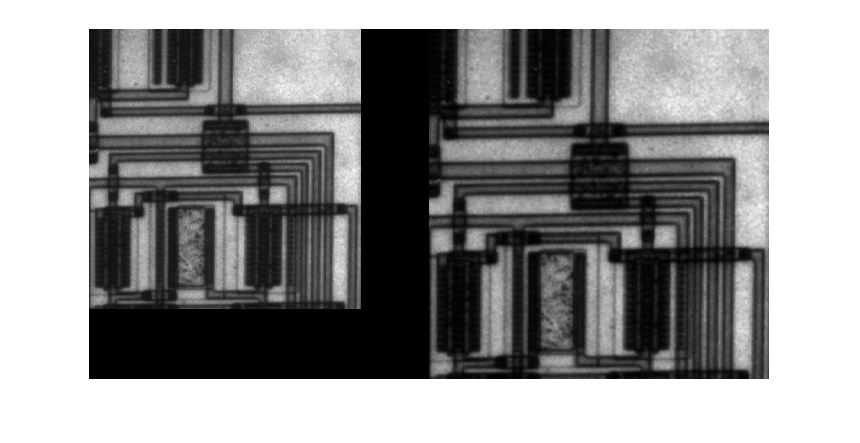本示例说明如何使用imresize函数调整图像大小。
指定放大倍数
将图像读入工作区。

 I = imread('circuit.tif');

使用imresize功能调整图像尺寸。在此示例中，您指定放大倍数。要放大图像，请指定大于1的放大倍数。

 J = imresize(I,1.25);

在放大的版本旁边显示原始图像。

 figure
imshowpair(I,J,'montage')
axis off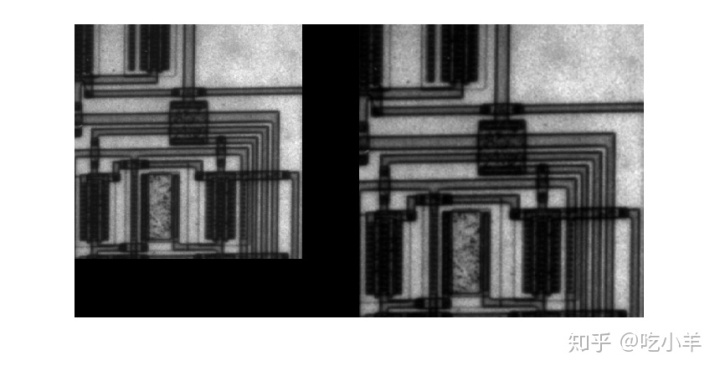指定输出图像的尺寸
再次调整图像大小，这次指定输出图像的所需大小，而不是放大值。传递给imresize函数一个包含输出图像中的行数和列数的向量。如果指定的尺寸不能产生与输入图像相同的纵横比，则输出图像将失真。如果将向量中的元素之一指定为NaN，imresize则将计算该尺寸的值以保留图像的纵横比。要执行多分辨率处理所需的调整大小操作，请使用impyramid。

 K = imresize(I,[100 150]);
figure, imshow(K)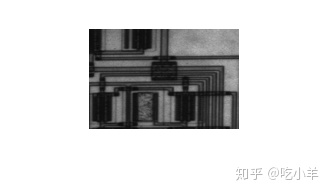指定插值方法
再次调整图像大小，这次指定插值方法。放大图像时，输出图像比原始图像包含更多的像素。imresize使用插值法确定这些像素的值，计算像素位置附近某组像素的加权平均值。imresize权重基于每个像素距该点的距离。默认情况下，imresize使用双三次插值，但是您可以指定其他插值方法或插值内核。有关imresize完整列表，请参见参考页。您还可以指定自己的自定义插值内核。本示例使用双线性插值。

 L = imresize(I,1.5,'bilinear');
figure, imshow(L)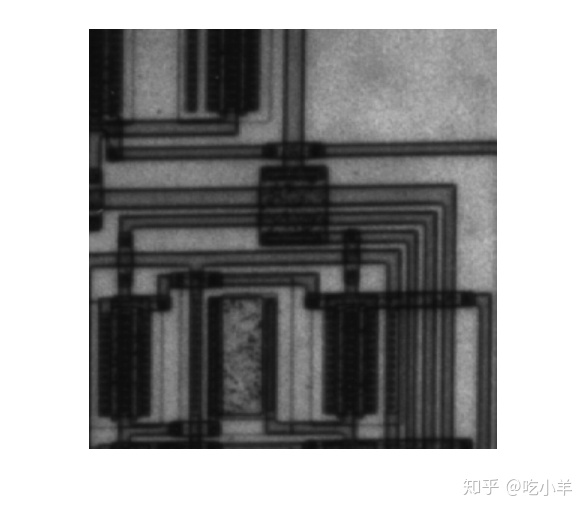缩小图像时防止混叠
再次调整图像大小，这次缩小图像。减小图像尺寸时，会丢失一些原始像素，因为输出图像中的像素较少。这可能会引入缺陷，例如混叠。由于尺寸减小而发生的混叠通常显示为阶梯图案（特别是在高对比度图像中），或者在输出图像中显示为莫尔（波纹效果）图案。默认情况下，imresize对于所有插值类型（最近邻除外），都使用抗混叠来限制锯齿对输出图像的影响。要关闭抗混叠功能，请指定“抗混叠”参数并将其值设置为false。即使启用了抗混叠功能，调整大小也会引入伪影，因为当您减小图像尺寸时，信息总是会丢失。

 M = imresize(I,.75,'Antialiasing',false);
figure, imshow(M)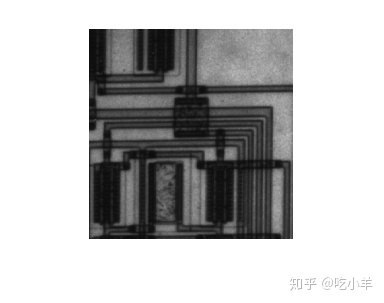注：本文根据MATLAB官网内容修改而成。
欢迎您进一步了解以下MATLAB系列文章：

吃小羊：MATLAB作图实例：00：索引​zhuanlan.zhihu.com吃小羊：MATLAB金融工具箱：00：索引​zhuanlan.zhihu.com展开全文• ## Matlab——图像缩放（插值法）

万次阅读 多人点赞 2018-07-30 15:49:36
输入原图像以及缩放图像的像素要求（宽度*高度），处理后输出新图像。  我是用matlab来实现scale(input_img,scale_size)函数的，输入图像路径以及要求实现的宽度scale_w和高度值scale_h即可。通过matlab把原...
实验内容：
用双线性内插法实现位深度为8的灰度图像的缩放。

思路：
输入原图像以及缩放后图像的像素要求（宽度*高度），处理后输出新图像。
我是用matlab来实现scale(input_img,scale_size)函数的，输入图像路径以及要求实现的宽度scale_w和高度值scale_h即可。通过matlab把原图像转化为img矩阵，这样矩阵img(a, b)元素的值就是原图像高度为a，宽度为b处的像素值。
设处理前后图像的宽度和高度分别为w、h，scale_w、scale_h。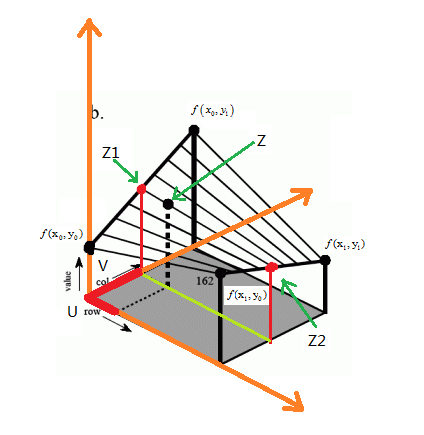如上图所示，row对应的是高度，设为x轴；col对应的是宽度，设为y轴；value对应的是灰度值，设为z轴。

通过缩放比例，可以求得新图像B在(i,j)处对应原图像的点为(x,y)（其中x= i*h/scale_h，y= j*w/scale_w）。
但是这两个值可能为浮点数，而像素中的位置是整数，所以这个点在原图像中对应的可能是“虚”点。所以，我们需
要根据找该“虚”点周围的四个点来进行双线性插值得到新图像的灰度值。我们应该取的是(x,y)邻近的四个像素
(x0,y0) 、(x0,y1) 、(x1,y0) 、(x1,y1)，它们对应的灰度值为f(x0,y0) 、f(x0,y1)、 f(x1,y0)、 f(x1,y1)。

先对x方向进行插值，再对y方向进行插值，计算如下：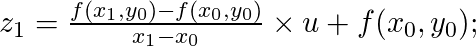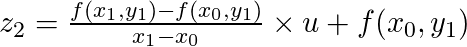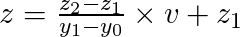反过来，先对y方向：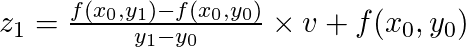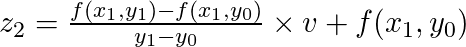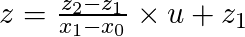显然，邻近像素的x1-x0 = y1-y0 = 1     整理式子得到：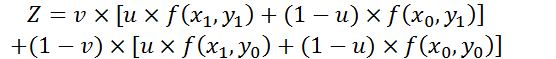这里u、v分别是x、y的小数部分，即u = x - x0; v = y - y0
要注意的是：上述公式img(a,b)中的a和b不能小于1，所以当x和y小于1时需要将其重新赋值为1。

代码：
function output_img = scale(input_img, scale_size)
%Input - input_img is a two-dimensional matrices storing image
%      - scale_size is a tuple of [width, height] defining the spatial resolution of output
%Output - output_img is the same as input_img

[h,w] = size(img); %获取行和列，即原图的高度和宽度

scale_w = scale_size(1); %根据输入获得缩放后的新宽度
scale_h = scale_size(2); %根据输入获得缩放后的新高度
output_img = zeros(scale_h, scale_w); %初始化

for i = 1 : scale_h         %缩放后的图像的（i,j）位置对应原图的（x,y）
for j = 1 : scale_w
x = i * h / scale_h;
y = j * w / scale_w;
u = x - floor(x);
v = y - floor(y); %取小数部分

if x < 1           %边界处理
x = 1;
end

if y < 1
y = 1;
end

%用原图的四个真实像素点来双线性插值获得“虚”像素的像素值
output_img(i, j) = img(floor(x), floor(y)) * (1-u) * (1-v) + ...
img(floor(x), ceil(y)) * (1-u) * v + ...
img(ceil(x), floor(y)) * u * (1-v) + ...
img(ceil(x), ceil(y)) * u * v;
end
end

imwrite(uint8(output_img), '../output_img.png'); %保存处理后的图像
imshow(input_img); %显示原图
figure,imshow(uint8(output_img)) %显示处理后的图像

运行时若图片在代码上一级目录，则输入scale('../a.png', [450,300]);

效果图：
原图为384*256：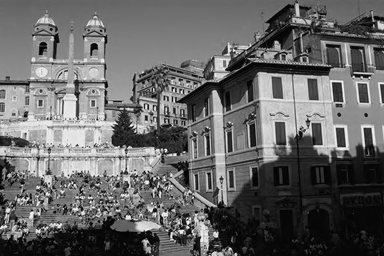缩小成192*128：放大成450*300：缩放成500*200：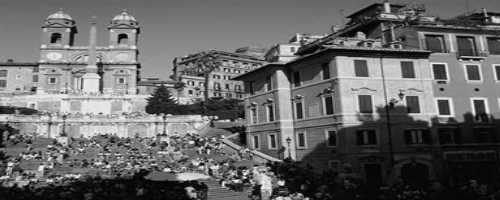//
转载：https://blog.csdn.net/Stella_Chan/article/details/78025020?locationNum=5&fps=1
展开全文插值法
• Matlab图像缩放 Matlab实现函数代码如下： clc I=rgb2gray(imread('Campus-scenery2sk.JPG')); figure,imshow(I); title('原图'); reduceI=imresize(I,0.5); %原图像I缩小0.5倍 figure,imshow(reduceI); title('...
• colorbar 显示彩条 getimage 由坐标轴得到图像数据 ice（DIPUM） 交互彩色编辑 ...imagesc 缩放数据并显示为图像 immovie 由多帧图像制作电影 imshow 显示图像 imview 在Image Viewer显示图像 montag...
• matlab实现图像缩放 缩放同样是仿射变换的一种特例，它接受水平和垂直两个方向的缩放值。 matlab 实现水平，垂直，水平垂直缩放： clear all close all clc img = imread('test.jpg'); %读取输入图片的...
• ## matlab里的图像处理函数

万次阅读 多人点赞 2019-02-19 15:56:54
一、imfinfo函数——查看图像文件信息，注意参数是文件路径和文件名，不是图像对应的矩阵。 &gt;&gt; imfinfo('C:\Users\zhulf\Desktop\1.jpg') ans =   Filename: 'C:\Users\zhulf\Desktop\1.jpg'  ...imshow
• 将 RGB 图像读取到工作区。 RGB = imread('peppers.png'); 将 RGB 图像的大小调整为 64 行。imresize 会自动计算列数。 RGB2 = imresize(RGB, [64 NaN]);...imfinfo - 有关图形文件的信息, 此 MATLAB 函数计算机视觉
• 对BMP图像进行放大及旋转的操作，实现图像成倍数的放大，并在水平面上进行任意角度的旋转。
• 原文地址：Matlab图像处理函数汇总 作者：mimi 图像的变换  ① fft2：fft2函数用于数字图像的二维傅立叶变换，如：i=imread('104_8.tif'); j=fft2(i); ②ifft2:：ifft2函数用于数字图像的二维傅立叶反变换，如...编程 数据
• 参考：https://www.cnblogs.com/zangyu/p/5395504.html对图像进行固定大小的缩放clear; clc; ％清除以前的数据 folderName = dir('train'); ％显示train文件夹下的文件和文件夹 for i = 1 : length(folderNa...
• 1. 离散傅立叶变换的 Matlab实现Matlab 函数 fft、fft2 和 fftn 分别可以实现一维、二维和 N 维 DFT 算法；而函数 ifft、ifft2 和 ifftn 则用来计算反 DFT 。这些函数的调用格式如下：A＝fft(X,N,DIM)其中，X 表示...
• matlab缩放函数图像代码单发MultiBox检测器 注意：此存储库不再维护。 对于主动维护的易于使用的物体检测器，我建议考虑使用PyTorch Mask R-CNN。 该目录包含用于训练和评估本文所述的SSD对象检测器的代码： SSD: ...
• 以灰度图像circuit.tif为例，利用Matlab图像处理工具箱的imresize函数对图像进行比例缩放变换。要求：创建4个figure窗口（不可以用subplot，显示不出来放大效果），分别用于显示原始图像、等比例放大1.5倍后的图像...
• MATLAB图像处理工具箱提供了3种插值方法：第一种是最近邻插值（nearest neighbor interpolation），最近邻插值的输出像素值等于输入图像与其最临近的像素点的值；第二种是双线性插值（bilinear interpolation），...
• 计算机图像处理 i=imread'D:\ 123.bmp; %读一幅图像 j=imrotate(i,30%图像旋转30度 k=imresize(i,2%图像放大两倍 t=imresize(i,2'bilinear%采用双线性插值法进行放大两倍 m=imresize(i,0.8%图像缩小到0.8倍 p=...
• 处理图像过程一定要对图像类型进行转换，不然中间会出现很多操作错误，所以要把这部分的内容进行学习整理一下，有哪些函数可以更便捷的转换呢！ 图像类型转换关系如下所示 RGB转灰度 rgb2gray() X = rgb2gray...
• 图像缩放函数： J = imresize ( I , rate , method ) ; 1 其中，I为原图像矩阵；rate为缩放比例，大于1为放大，小于1为缩小；method为选择插值方法，默认为’nearest’（最近邻插值），还有’bilinear’...
• 目录图像平移、旋转、缩放、镜像的MATLAB实现（仿照MATLAB内置函数实现）原理代码平移镜像...（《数字图像处理》课程实验3） 参考MATLAB内置im系列函数实现。本次实验结果上没有做到与内置函数100%一致，但相差无几...
• 经典图像/视频缩放算法原理及MATLAB\Simulink实现1 图像/视频缩放介绍2 图像缩放的经典算法2.1 最近邻插值法2.2 双线性插值法3 图像缩放算法的MATLAB实现3.1 最近邻算法实现3.2 双线性插值算法实现4 视频无极缩放的...
• matlab实现图像缩放、旋转、金字塔建立，自己写的代码，没有用到MATLAB中的相关函数，可实现任意角度及任意级别的金字塔和影像缩放
• matlab缩放函数图像代码超赞的超分辨率 精选的超高分辨率资源列表。 最近我们发布了对社区。 在本次调查，我们从不同方面回顾了该任务，包括问题陈述，数据集，评估指标，方法论和特定领域的应用程序。 具体来说，...
• 实验一 MATLAB图像处理实验报告 实 验 报告 课程名称 何变换 数字图像处理 实验名称 图像的几 姓 名 吴征宇 学 号 3101110002 专业班级 实验日期 20XX 年10 月 18日 成绩 指导教师 实验目的 掌握图像平移缩放旋转与...
• matlab缩放函数图像代码清除 评估多目标跟踪算法的标准度量标准是CLEAR MOT。 该度量标准在论文进行了描述。 使用我们的代码的论文  D. Karatzas，F。Shafait，S。Uchida，M。Iwamura，L。Gomez i Bigorda，......

# matlab中图像缩放的函数matlab 订阅